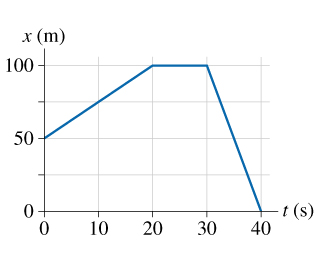# Problem: a) What is the bicyclist's velocity at t=10s?Express your answer using two significant figures.b) What is the bicyclist's velocity at t=25s?Express your answer using two significant figures.c) What is the bicyclist's velocity at t=35s?Express your answer using two significant figures.

###### FREE Expert Solution

From a position-time graph, velocity is:

$\overline{){\mathbf{v}}{\mathbf{=}}\frac{\mathbf{∆}\mathbf{x}}{\mathbf{∆}\mathbf{t}}}$

This means that velocity is the slope of the position-time graph.

a)

At t = 10s, the motion is in the interval, t = 0s and t = 20s

98% (169 ratings)###### Problem Detailsa) What is the bicyclist's velocity at t=10s?

b) What is the bicyclist's velocity at t=25s?

c) What is the bicyclist's velocity at t=35s?